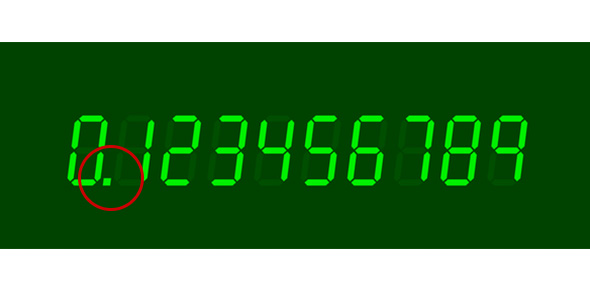# Multiplying Decimals Practice Vida

Approved & Edited by ProProfs Editorial Team
The editorial team at ProProfs Quizzes consists of a select group of subject experts, trivia writers, and quiz masters who have authored over 10,000 quizzes taken by more than 100 million users. This team includes our in-house seasoned quiz moderators and subject matter experts. Our editorial experts, spread across the world, are rigorously trained using our comprehensive guidelines to ensure that you receive the highest quality quizzes.
| By Annettewilson
A
Annettewilson
Community Contributor
Quizzes Created: 4 | Total Attempts: 923
Questions: 10 | Attempts: 389SettingsPlease fill in the answer below. You MUST show all work on the worksheet and turn in or your score will go in the gradebook as a zero.

• 1.

### 12.10 x 5 =

Explanation
The correct answer is 60.50, 60.5 because when you multiply 12.10 by 5, you get a product of 60.50. Additionally, 60.5 is the same value rounded to one decimal place.

Rate this question:

• 2.

### 17.05 x 2

Explanation
The given expression is a multiplication of 17.05 and 2. When we multiply 17.05 with 2, we get the result as 34.10. However, in decimal representation, trailing zeroes after the decimal point are insignificant. Therefore, the answer can also be written as 34.1. Both 34.1 and 34.10 represent the same value.

Rate this question:

• 3.

### 16.23 x 3

Explanation
The given expression is a multiplication problem. To find the product of 16.23 and 3, we multiply the two numbers. Multiplying 16.23 by 3 gives us the result of 48.69.

Rate this question:

• 4.

### 2.72 x 9

Explanation
The given expression, 2.72 x 9, can be solved by multiplying 2.72 by 9. The product of these two numbers is 24.48.

Rate this question:

• 5.

### 1.59 x 6

Explanation
The given expression is a multiplication problem, where 1.59 is multiplied by 6. When we multiply 1.59 by 6, we get the result of 9.54.

Rate this question:

• 6.

### 1.75 x 5

Explanation
The given expression is a multiplication problem. To find the product of 1.75 and 5, we multiply 1.75 by 5. The result of this multiplication is 8.75.

Rate this question:

• 7.

### 2.50 x 1.5

Explanation
When multiplying 2.50 by 1.5, the result is 3.75. This can also be expressed as 3.750 when written with three decimal places. Both answers are correct and represent the product of the two numbers.

Rate this question:

• 8.

### 15.79 x 2.5

Explanation
The given question is asking for the product of 15.79 and 2.5. When these two numbers are multiplied, the result is 39.475.

Rate this question:

• 9.

### 3.72 x 1.4

Explanation
The given question asks for the product of 3.72 and 1.4. When these two numbers are multiplied, the result is 5.208.

Rate this question:

• 10.

### 16.37 x 1.5

Related TopicsBack to top